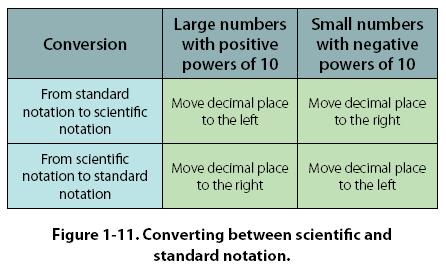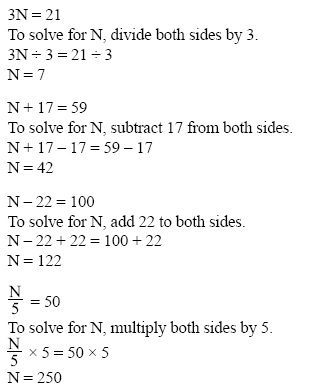Converting Numbers from Standard Notation to Scientific Notation Example: Convert 1,244,000,000,000 to scientific notation as follows. First, note that the decimal point is to the right of the last zero. (Even though it is not usually written, it is assumed to be there.) 1,244,000,000,000 = 1,244,000,000,000 To change to the format of scientific notation, the decimal point must be moved to the position between the first and second digits, which in this case is between the 1 and the 2. Since the decimal point must be moved 12 places to the left to get there, the power of 10 will be 12. Remember that large numbers always have a positive exponent. Therefore, 1,244,000,000,000 = 1.244 x 1012 when written in scientific notation. Example: Convert 0.000000457 from standard notation to scientific notation. To change to the format of scientific notation, the decimal point must be moved to the position between the first and second numbers, which in this case is between the 4 and the 5. Since the decimal point must be moved 7 places to the right to get there, the power of 10 will be -7. Remember that small numbers (those less than one) will have a negative exponent. Therefore, 0.000000457 = 4.57 x 10-7 when written in scientific notation. Converting Numbers from Scientific Notation to Standard Notation Example: Convert 3.68 x 107 from scientific notation to standard notation, as follows. To convert from scientific notation to standard notation, move the decimal place 7 places to the right. 3.68 x 107 = 36800000 = 36,800,000. Another way to think about the conversion is 3.68 x 107 = 3.68 x 10,000,000 = 36,800,000. Example: Convert 7.1543 x 10-10 from scientific notation to standard notation. Move the decimal place 10 places to the left: 7.1543 x 10-10 =.00000000071543. Another way to think about the conversion is 7.1543 x 10-10 = 7.1543 x .0000000001 = .00000000071543 When converting, remember that large numbers always have positive powers of ten and small numbers always have negative powers of ten. Refer to Figure 1-11 to determine which direction to move the decimal point.Addition, Subtraction, Multiplication, and Division of Scientific Numbers To add, subtract, multiply, or divide numbers in scientific notation, change the scientific notation number back to standard notation. Then add, subtract, multiply or divide the standard notation numbers. After the computation, change the final standard notation number back to scientific notation. Algebra Algebra is the branch of mathematics that uses letters or symbols to represent variables in formulas and equations. For example, in the equation D = V x T, where Distance = Velocity x Time, the variables are: D, V, and T. Equations Algebraic equations are frequently used in aviation to show the relationship between two or more variables. Equations normally have an equals sign (=) in the expression. Example: The formula A = p x r2 shows the relationship between the area of a circle (A) and the length of the radius (r) of the circle. The area of a circle is equal to p (3.1416) times the radius squared. Therefore, the larger the radius, the larger the area of the circle. Algebraic Rules When solving for a variable in an equation, you can add, subtract, multiply or divide the terms in the equation, you do the same to both sides of the equals sign. Examples: Solve the following equations for the value N.©AvStop Online Magazine                                                                                                                                                      Contact Us              Return To Books